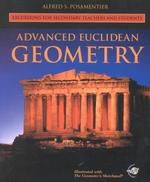Advanced Euclidean Geometry : Excursions for Secondary Teachers and Students （PAP/CDR）

• ただいまウェブストアではご注文を受け付けておりません。 ⇒古書を探す
• 製本 Paperback:紙装版/ペーパーバック版／ページ数 254 p.
• 言語 ENG,ENG
• 商品コード 9781930190856
• DDC分類 516.22

Preface                                            v
Introduction vii
About the Author ix
Elementary Euclidean Geometry Revisited 1 (23)
Review of Basic Concepts of Geometry 2 (11)
Learning from Geometric Fallacies 13 (7)
Common Nomenclature 20 (4)
Concurrency of Lines in a Triangle 24 (18)
Introduction 25 (2)
Ceva's Theorem 27 (5)
Applications of Ceva's Theorem 32 (4)
The Gergonne Point 36 (6)
Collinearity of Points 42 (35)
Duality 43 (2)
Menelaus's Theorem 45 (3)
Applications of Menelaus's Theorem 48 (3)
Desargues's Theorem 51 (3)
Pascal's Theorem 54 (3)
Brianchon's Theorem 57 (3)
Pappus's Theorem 60 (1)
The Simson Line 61 (7)
Radical Axes 68 (9)
Some Symmetric Points in a Triangle 77 (10)
Introduction 78 (1)
Equiangular Point 79 (3)
A Property of Equilateral Triangles 82 (1)
A Minimum Distance Point 83 (4)
More Triangle Properties 87 (29)
Introduction 88 (1)
Angle Bisectors 88 (8)
Stewart's Theorem 96 (4)
Miquel's Theorem 100(6)
Medians 106(10)
Centers of a Quadrilateral 119(4)
Ptolemy's Theorem 128(4)
Applications of Ptolemy's Theorem 132(10)
Equicircles 142(15)
Points of Tangency 143(5)
The Nine-Point Circle 157(15)
Introduction to the Nine-Point Circle 158(5)
Altitudes 163(5)
The Nine-Point Circle Revisited 168(4)
Triangle Constructions 172(28)
Introduction 173(7)
Selected Constructions 180(20)
Circle Constructions 200(15)
Introduction 201(1)
The Problem of Apollonius 201(14)
The Golden Section and Fibonacci Numbers 215(34)
The Golden Ratio 216(4)
Fibonacci Numbers 220(6)
Lucas Numbers 226(4)
Fibonacci Numbers and Lucas Numbers in 230(5)
Geometry
The Golden Rectangle Revisited 235(5)
The Golden Triangle 240(9)
Index 249